Question

# in C++ You are to define a structure containing the id number, three test scores, and...

in C++ You are to define a structure containing the id number, three test scores, and their average, for each student in a class of 20. The file pr2data.txt is included and contains the data. The program is to include the following:

1. A function that reads 20 records of data from the file, finds the average for each student and prints each student’s record.
2. A function that lists the ids and averages for all the students whose average is above 70.00.
3. A function that displays the ids and averages that are greater than or equals to 85.00. to do that it calls a sort function that sorts the arrays in ascending order based on the averages and calls a search function that uses a binary search to return the position if found. Make sure you handle multiple values of 85.00 if any.
4. A function that sorts the array, in ascending order, based on id, using the selection sort (procedure is in the book). In the function you need to place a counter to keep the number of swaps it took before the array became sorted.
5. Perform a binary search that will display the id, test1, test2, test3 and average for a requested id, this should repeat until the user enters ‘n’ or ‘N’ in response to a prompt.

pr2data.txt

1234 52   70 75
2134 90   75 90
3124 90   95 98
4532 11   17 81
5678 20   12 45
6134 34   45 55
7874 60   100 56
8026 70   10 66
9893 34   9 77
2233 78   20 78
1947 45   40 88
3456 78   55 78
2877 55   50 99
3189 87   94 74
2132 75   100 80
4602 89   50 91
3445 78   60 78
5405 11   11 0
4556 78   20 10
6999 88   98 89

Please let me know if anything is required.

Copyable code:

#include <iostream>
#include <fstream>
#include <sstream>
#include <string>
using namespace std;

//the structure to store the file contents
struct Student_Records {
int id;
int score1;
int score2;
int score3;
};

//function ti calculate hte student Average
float findstavg(int q1,int q2,int q3)
{
return (q1+q2+q3)/3.0;
}

//function to find the high score
int findhigh(int q[],int n)
{
int max =q;
for(int i=1;i<n;i++)
{
if(q[i]>max) //condition for max
{
max=q[i];
}
}

return max;
}

//function to find the low score
int findlow(int q[],int n)
{
int min =q;
for(int i=1;i<n;i++)
{
if(q[i]<min)//condition for min
{
min=q[i];
}
}

return min;
}

//function to find the quiz average
float findqzavg(int q[],int n)
{
int s=0;
for(int i=0;i<n;i++)
{
s+=q[i]; //calculating the sum
}

double avg = s/(n*3.0); //calculating the avg
return avg;
}

int main()
{

string line;
ifstream myfile("pr2data.txt"); //opening the input file
ifstream myfile1("pr2data.txt");

//program to find the number of lines are present in the file for declaring the array sozes
int linecount=0;

//condition to check whether able to open the file or not
if (myfile.is_open())
{
while ( getline (myfile,line) )
{
linecount++;

}

myfile.close();
}

else {cout << "Error, the file does not exist\n"; }

struct Student_Records student_array[linecount];
//code to perform the required actions
if (myfile1.is_open())
{

ofstream outfile;
outfile.open("output.txt"); //output file to write the contents
outfile<<"Student Quiz1 Quiz2 Quiz3 Average\n";

//arrays to store the values
int student[linecount];
int quiz1[linecount];
int quiz2[linecount];
int quiz3[linecount];

int j=0;
while ( getline (myfile1,line) )
{
outfile<<line;//writintg the contents to the output file
int s;
string delimiter = " "; //splitting the line with spaces

int i=0;
size_t pos = 0;
string token;
while ((pos = line.find(delimiter)) != string::npos)
{
token = line.substr(0, pos);

stringstream geek(token); //converting the string to int
int x = 0;
geek >> x;
s[i]=x;
i++;
line.erase(0, pos + delimiter.length());
}

//converting the string to int
token = line;
stringstream geek(token);
int x = 0;
geek >> x;
s[i]=x;

//assigning the values into the structure
student_array[j].id=s;
student_array[j].score1=s;
student_array[j].score2=s;
student_array[j].score3=s;

student[j]=student_array[j].id;
quiz1[j]=student_array[j].score1;
quiz2[j]=student_array[j].score2;
quiz3[j]=student_array[j].score3;

//calling the student average function
float std_avg = findstavg(quiz1[j],quiz2[j],quiz3[j]);
outfile<<" "<<std_avg<<"\n"; //writintg the student average details to the output file

j++;
}

int high_score_q1 = findhigh(quiz1,22);//function to find the high score values
int high_score_q2 = findhigh(quiz2,22);//function to find the high score values
int high_score_q3 = findhigh(quiz3,22);//function to find the high score values

//writintg the high score values of each quiz to the output file
outfile<<"High score "<<high_score_q1<<" "<<high_score_q2<<" "<<high_score_q3<<" \n";

int low_score_q1 = findlow(quiz1,22); //function to find the low score values
int low_score_q2 = findlow(quiz2,22); //function to find the low score values //function to find the low score values
int low_score_q3 = findlow(quiz3,22);

//writintg the low score values of each quiz to the output file
outfile<<"Low score "<<low_score_q1<<" "<<low_score_q2<<" "<<low_score_q3<<" \n";

float avg_score_q1 = findqzavg(quiz1,22);//function to find the quiz average values
float avg_score_q2 = findqzavg(quiz2,22);//function to find the quiz average values
float avg_score_q3 = findqzavg(quiz3,22);//function to find the quiz average values

//writintg the average quiz values of each quiz to the output file
outfile<<"Quiz Average "<<avg_score_q1<<" "<<avg_score_q2<<" "<<avg_score_q3<<" \n";

myfile.close();
}

return 0;
}

Inputfile screenshot :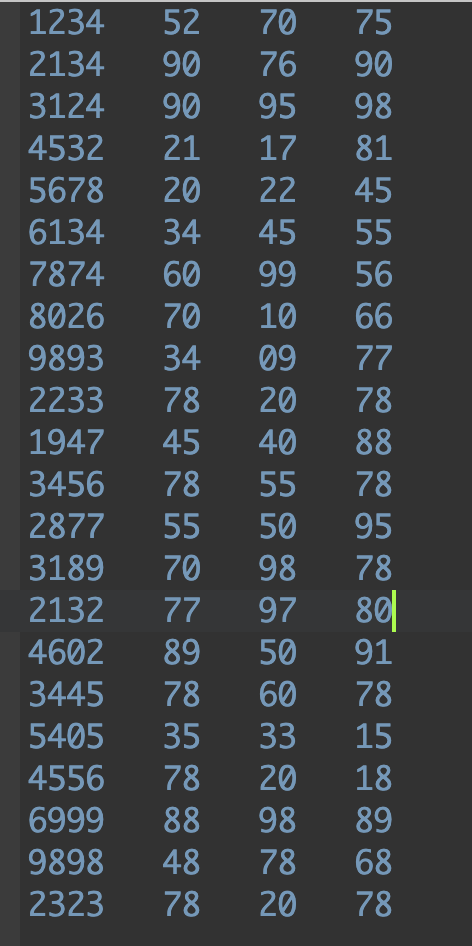output screenshot :

Testcase1 :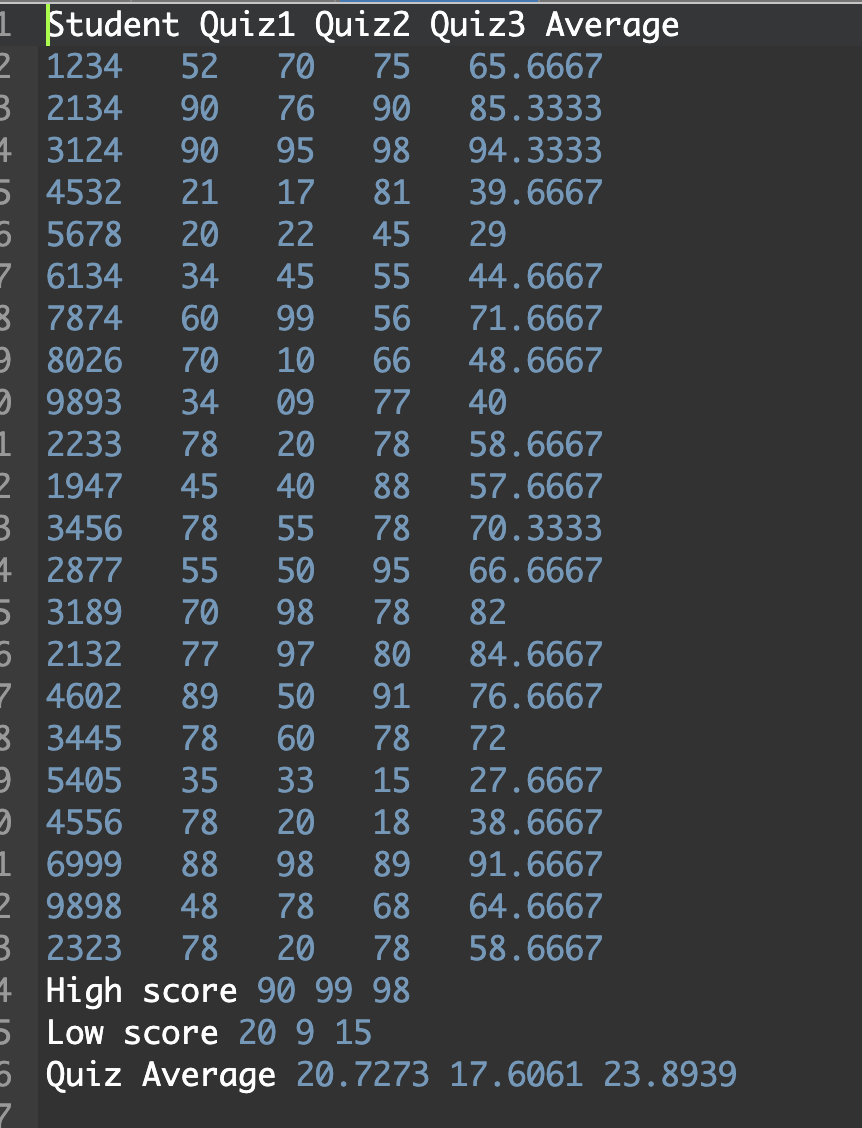Testcase 2:

I gave the file name as pr2dat.txt instead of pr2data.txt so we should get an error as the input file is not exist, attaching the screenshot for the same.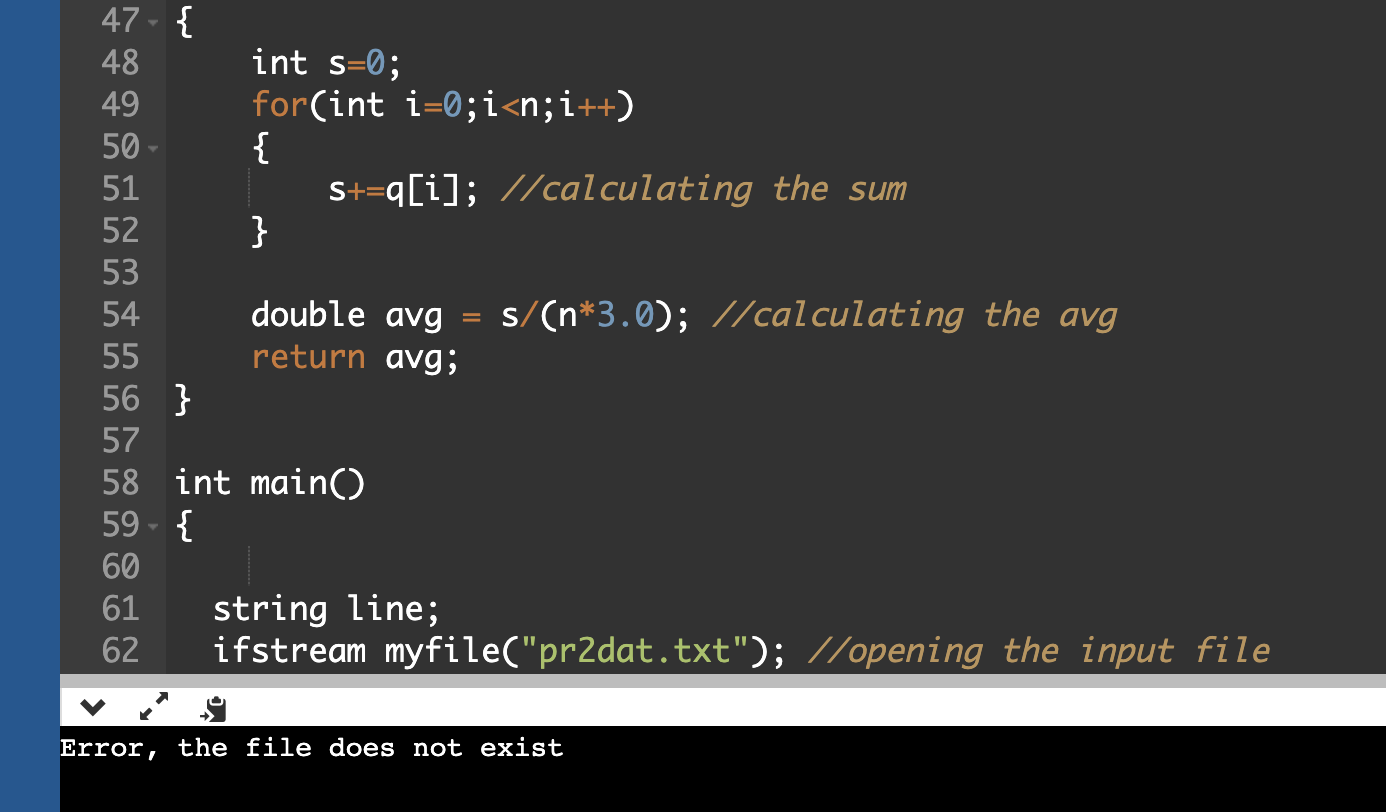#### Earn Coins

Coins can be redeemed for fabulous gifts.

Similar Homework Help Questions
• ### C++ program that reads students' names followed by their test scores (5 scores) from the given...

C++ program that reads students' names followed by their test scores (5 scores) from the given input file, students.txt. The program should output to a file, output.txt, each student's first name, last name, followed by the test scores and the relevant grade. All data should be separated by a single space. Student data should be stored in a struct variable of type StudentType, which has four components: studentFName and studentLName of type string, an array of testScores of type int...

• ### C++ Sorting and Searching 1. Mark the following statements as true or false. a. A sequential...

C++ Sorting and Searching 1. Mark the following statements as true or false. a. A sequential search of a list assumes that the list elements are sorted in ascending order. b. A binary search of a list assumes that the list is sorted. 2. Consider the following list: 63 45 32 98 46 57 28 100 Using a sequential search, how many comparisons are required to determine whether the following items are in the list or not? (Recall that comparisons...

Please help me with this program.You are to write a C++ program that will read in up to 15 students with student information and grades. Your program will compute an average and print out certain reports. Format: The information for each student is on separate lines of input. The first data will be the student�s ID number, next line is the students name, next the students classification, and the last line are the 10 grades where the last grade is...

• ### Consider the below matrixA, which you can copy and paste directly into Matlab.

Problem #1: Consider the below matrix A, which you can copy and paste directly into Matlab. The matrix contains 3 columns. The first column consists of Test #1 marks, the second column is Test # 2 marks, and the third column is final exam marks for a large linear algebra course. Each row represents a particular student.A = [36 45 75 81 59 73 77 73 73 65 72 78 65 55 83 73 57 78 84 31 60 83...

• ### 1. Forecast demand for Year 4. a. Explain what technique you utilized to forecast your demand....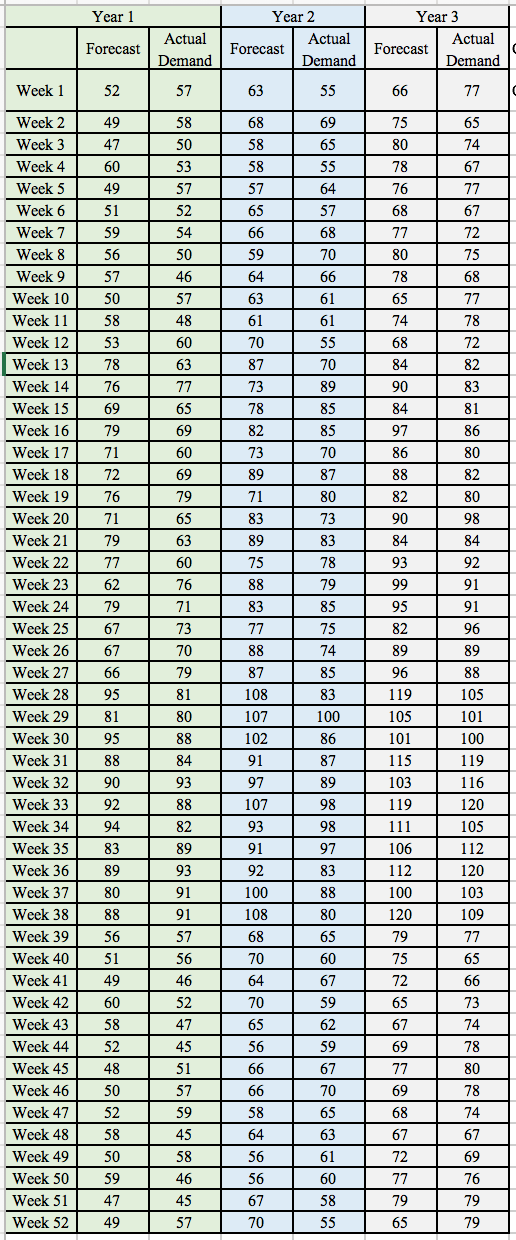1. Forecast demand for Year 4. a. Explain what technique you utilized to forecast your demand. b. Explain why you chose this technique over others. Year 3 Year 1 Year 2 Actual Actual Actual Forecast Forecast Forecast Demand Demand Demand Week 1 52 57 63 55 66 77 Week 2 49 58 68 69 75 65 Week 3 47 50 58 65 80 74 Week 4 60 53 58 55 78 67 57 Week 5 49 57 64 76 77...

• ### Write a main function that declares the names, marks, number of elements as well as the...

Write a main function that declares the names, marks, number of elements as well as the value to be searched for and the index of the returned function calls. Read and display the contents of names and marks. Ask the user for a name and using the linear search return the index to the user. If -1 is returned then the name is not in the file. Otherwise write out the name and mark for that student. Sort the arrays...

• ### Your IT department provided you data on patients that received ER services, their GHHS, and their recovery time. Prepare a report to share with the owners of the facility that will help you make infor...

Your IT department provided you data on patients that received ER services, their GHHS, and their recovery time. Prepare a report to share with the owners of the facility that will help you make informed decisions about how long you can expect a patients’ recovery time would be based on their GHHS. Based on your findings provide recommendations on your plan moving forward to improve the functioning of your facilities in generating revenue. Prepare a report that addresses each of...

• ### Must be in Python 3 exercise_2.py # Define the Student class class Student():    # Ini...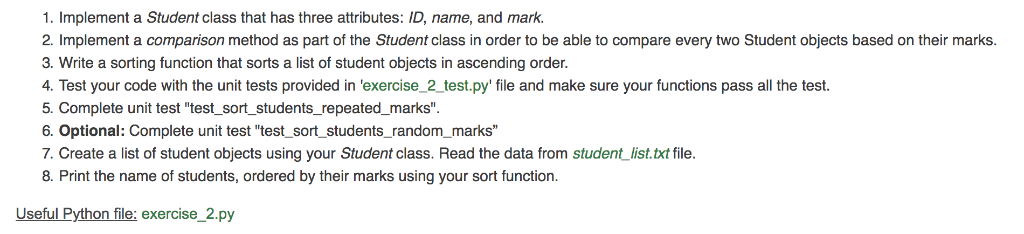Must be in Python 3 exercise_2.py # Define the Student class class Student():    # Initialize the object properties def __init__(self, id, name, mark): # TODO # Print the object as an string def __str__(self): return ' - {}, {}, {}'.format(self.id, self.name, self.mark) # Check if the mark of the input student is greater than the student object # The output is either True or False def is_greater_than(self, another_student): # TODO # Sort the student_list # The output is the sorted...

• ### == Programming Assignment == For this assignment you will write a program that controls a set...

== Programming Assignment == For this assignment you will write a program that controls a set of rovers and sends them commands to navigate on the Martian surface where they take samples. Each rover performs several missions and each mission follows the same sequence: deploy, perform one or more moves and scans, then return to base and report the results. While on a mission each rover needs to remember the scan results, in the same order as they were taken,...

• ### Using C programming For this project, you have been tasked to read a text file with student grade...

Using C programming For this project, you have been tasked to read a text file with student grades and perform several operations with them. First, you must read the file, loading the student records. Each record (line) contains the student’s identification number and then four of the student’s numerical test grades. Your application should find the average of the four grades and insert them into the same array as the id number and four grades. I suggest using a 5th...